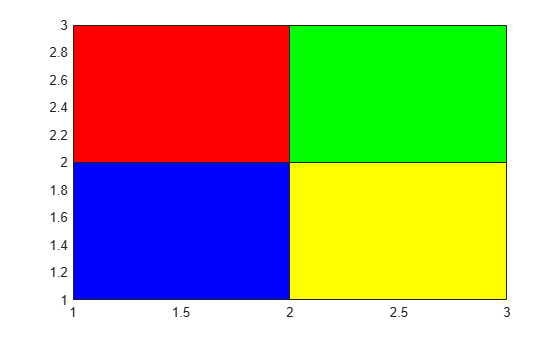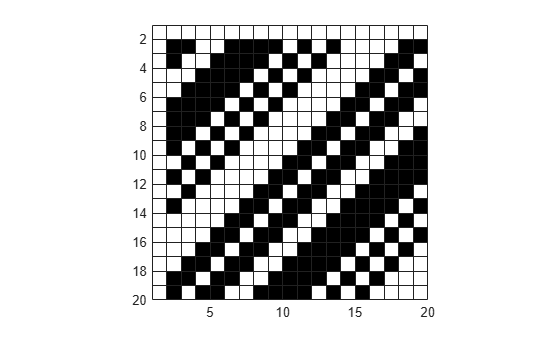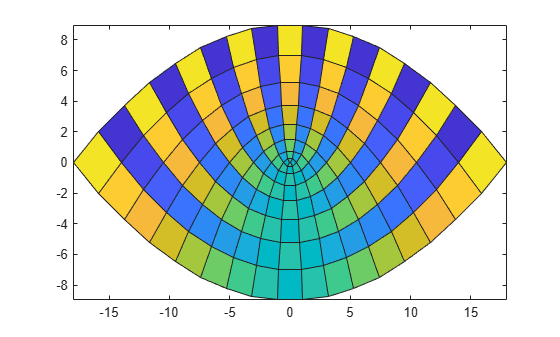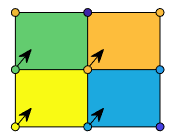# pcolor

Pseudocolor plot

•## Syntax

``pcolor(C)``
``pcolor(X,Y,C)``
``pcolor(ax,___)``
``s = pcolor(___)``

## Description

example

````pcolor(C)` creates a pseudocolor plot using the values in matrix `C`. A pseudocolor plot displays matrix data as an array of colored cells (known as faces). MATLAB® creates this plot as a flat surface in the x-y plane. The surface is defined by a grid of x- and y-coordinates that correspond to the corners (or vertices) of the faces. The grid covers the region `X=1:n` and `Y=1:m`, where `[m,n] = size(C)`. Matrix `C` specifies the colors at the vertices. The color of each face depends on the color at one of its four surrounding vertices. Of the four vertices, the one that comes first in the x-y grid determines the color of the face.```

example

````pcolor(X,Y,C)` specifies the x- and y-coordinates for the vertices. The size of `C` must match the size of the x-y coordinate grid. For example, if `X` and `Y` define an m-by-n grid, then `C` must be an m-by-n matrix.```

example

````pcolor(ax,___)` specifies the target axes for the plot. Specify `ax` as the first argument in any of the previous syntaxes.```

example

````s = pcolor(___)` returns a `Surface` object. Use `s` to set properties on the plot after creating it. For a list of properties, see Surface Properties.```

## Examples

collapse all

Create coordinate vectors `X` and `Y` and a colormap called `mymap` containing five colors: red, green, blue, yellow, and black.

```X = [1 2 3; 1 2 3; 1 2 3]; Y = X'; mymap = [1 0 0; 0 1 0; 0 0 1; 1 1 0; 0 0 0];```

Create matrix `C` that maps the colormap colors to the nine vertices. Four of the nine vertices determine the colors of the faces. Specify the colors at those vertices to make the faces red (`1`), green (`2`), blue (`3`), and yellow (`4`), respectively. Set the colors at the other vertices to black (`5`).

`C = [3 4 5; 1 2 5; 5 5 5];`

Plot the faces, and call the `colormap` function to replace the default colormap with `mymap`.

```pcolor(X,Y,C) colormap(mymap)```A Hadamard matrix has elements that are either `1` or `-1`. A good way to visualize this matrix is with a two-color colormap.

Create a 20-by-20 Hadamard matrix. Then plot the matrix using a black and white colormap. Use the `axis` function to reverse the direction of the y-axis and set the axis lines to equal lengths.

```C = hadamard(20); pcolor(C) colormap(gray(2)) axis ij axis square```Create color matrix `C`. Then create a pseudocolor plot of `C`, and store the `Surface` object in the return argument `s`.

```C = [1 2 3; 4 5 6; 7 8 9]; s = pcolor(C);```Change the border color by setting the `EdgeColor` property of `s`. Make the border thicker by setting the `LineWidth` property.

```s.EdgeColor = [1 0.7 0.3]; s.LineWidth = 6;```Create color matrix `C`. Then create a pseudocolor plot of `C`, and store the `Surface` object in the return argument `s`.

```C = [5 13 9 7 12; 11 2 14 8 10; 6 1 3 4 15]; s = pcolor(C);```To interpolate the colors across the faces, set the `FaceColor` propery of `s` to `'interp'`.

`s.FaceColor = 'interp';`Create matrices `X` and `Y`, which define a regularly spaced grid of vertices. Calculate matrix `LY` as the log of `Y`. Then create matrix `C` containing alternating pairs of rows of color indices.

```[X,Y] = meshgrid(1:20); LY = log(Y); colorscale = [1:20; 20:-1:1]; C = repmat(colorscale,10,1);```

Plot `X` and `LY`, using the colors specified in `C`. Then adjust the tick labels on the y-axis.

```s = pcolor(X,LY,C); tickvals = LY(2:2:20,1)'; set(gca,'YTick',tickvals);```Create matrices `X` and `Y`, which define a regularly spaced grid of vertices. Calculate matrices `XX` and `YY` as functions of `X` and `Y`. Then create matrix `C` containing alternating pairs of rows of color indices.

```[X,Y] = meshgrid(-3:6/17:3); XX = 2*X.*Y; YY = X.^2 - Y.^2; colorscale = [1:18; 18:-1:1]; C = repmat(colorscale,9,1);```

Plot `XX` and `YY` using the colors in `C`.

`pcolor(XX,YY,C);`Starting in R2019b, you can display a tiling of plots using the `tiledlayout` and `nexttile` functions. Call the `tiledlayout` function to create a 1-by-2 tiled chart layout. Call the `nexttile` function to create the axes objects `ax1` and `ax2`. Create two pseudocolor plots by specifying the axes as the first argument to `pcolor`.

```tiledlayout(1,2) % Left plot ax1 = nexttile; C1 = rand(20,10); pcolor(ax1,C1) % Right plot ax2 = nexttile; C2 = rand(50,10); pcolor(ax2,C2)```## Input Arguments

collapse all

Color matrix containing indices into the colormap. The values in `C` map colors in the colormap array to the vertices surrounding each face. The color of a face depends on the color at one of its four vertices. Of the four vertices, the one that come first in `X` and `Y` determines the color of the face. If you do not specify `X` and `Y`, MATLAB uses `X=1:n` and `Y=1:m`, where `[m,n] = size(C)`. Because of this relationship between the vertex colors and face colors, none of the values in the last row and column of `C` are represented in the plot.Note

The first vertex of a face is the one that is closest to the upper-left corner of the corresponding matrix. However, because the y-axis increases from bottom to top, the first vertex shown in the plot is typically the one in the lower-left corner of the face. To get the effect you want, you might have to change the orientation of the y-axis or the orientation of matrix `C`.

The values in `C` scale to the full range of the colormap. The smallest value in `C` maps to the first row in the colormap array. The largest value in `C` maps to the last row in the colormap array. The intermediate values in `C` map linearly to the intermediate rows of the colormap array. You can adjust this mapping using the `caxis` function.

The `CData` property of the `Surface` object stores the values of `C`.

Data Types: `single` | `double` | `int8` | `int16` | `int32` | `int64` | `uint8` | `uint16` | `uint32` | `uint64`

x-coordinates, specified as a matrix the same size as `C`, or as a vector of length `n`, where `[m,n] = size(C)`. The default value of `X` is the vector `(1:n)`.

To create a rectangular grid of vertices, specify `X` as either of the following:

• A vector containing values that are increasing or decreasing.

• A matrix that is increasing or decreasing along one dimension and is constant along the other dimension. Set the dimension that varies to the opposite of the dimension that varies in matrix `Y`. You can use the `meshgrid` function to create the `X` and `Y` matrices.

To create a parametric grid, create a rectangular grid and pass it through a mathematical function.

Example: `X = 1:10`

Example: `X = [1 2 3; 1 2 3; 1 2 3]`

Example: `[X,Y] = meshgrid(1:10)`

The `XData` property of the `Surface` object stores the x-coordinates.

Data Types: `single` | `double` | `int8` | `int16` | `int32` | `int64` | `uint8` | `uint16` | `uint32` | `uint64` | `categorical` | `datetime` | `duration`

y-coordinates, specified as a matrix the same size as `C`, or as a vector of length `m`, where `[m,n] = size(C)`. The default value of `Y` is the vector `(1:m)`.

To create a rectangular grid of vertices, specify `Y` as either of the following:

• A vector containing values that are increasing or decreasing.

• A matrix that is increasing or decreasing along one dimension and is constant along the other dimension. Set the dimension that varies to the opposite of the dimension that varies in matrix `X`. You can use the `meshgrid` function to create the `X` and `Y` matrices.

To create a parametric grid, create a rectangular grid and pass it through a mathematical function.

Example: `Y = 1:10`

Example: `Y = [1 1 1; 2 2 2; 3 3 3]`

Example: `[X,Y] = meshgrid(1:10)`

The `YData` property of the `Surface` object stores the y-coordinates.

Data Types: `single` | `double` | `int8` | `int16` | `int32` | `int64` | `uint8` | `uint16` | `uint32` | `uint64` | `categorical` | `datetime` | `duration`

Target axes, specified as an `Axes` object. If you do not specify the axes, then `pcolor` plots into the current axes.

## Algorithms

Use the `pcolor`, `image`, and `imagesc` functions to display rectangular arrays of colored cells. The relationship between the color matrix `C` and the colored cells is different in each case.

• `pcolor(C)` uses the values in `C` to define the vertex colors by scaling the values to the full range of the colormap. The size of `C` determines the number of vertices. The values in `C` map colors from the current colormap to the vertices surrounding each cell.

• `image(C)` uses `C` to define the cell colors by mapping the values directly into the colormap. The size of `C` determines the number of cells.

• `imagesc(C)` uses `C` to define the cell colors by scaling the values to the full range of the colormap. The size of `C` determines the number of cells.

## Version History

Introduced before R2006a